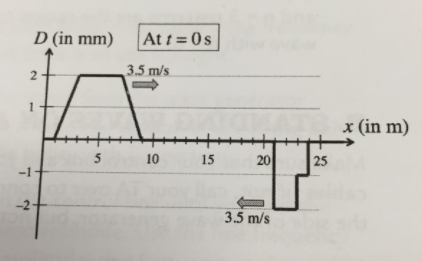# Problem: The graph on the right shows two waves at time t = 0 s, one moving toward the right at 3.5m/s and the other moving to the left at 3.5m/s. Draw the graphs of the waves at t = 1 s, t = 2 s, t = 3 s, and t = 4 s.

###### FREE Expert Solution

In this problem, we determine the position of the wave by using Δx = vt

89% (68 ratings)###### Problem Details

The graph on the right shows two waves at time t = 0 s, one moving toward the right at 3.5m/s and the other moving to the left at 3.5m/s. Draw the graphs of the waves at t = 1 s, t = 2 s, t = 3 s, and t = 4 s.